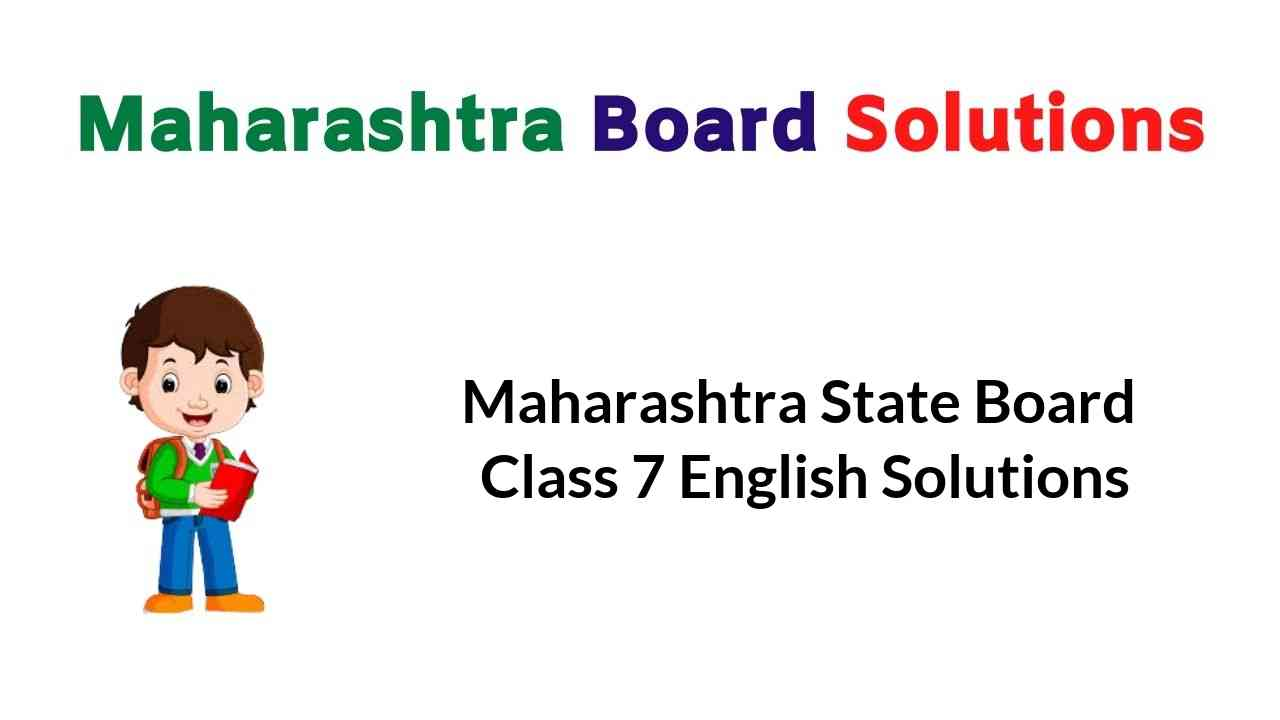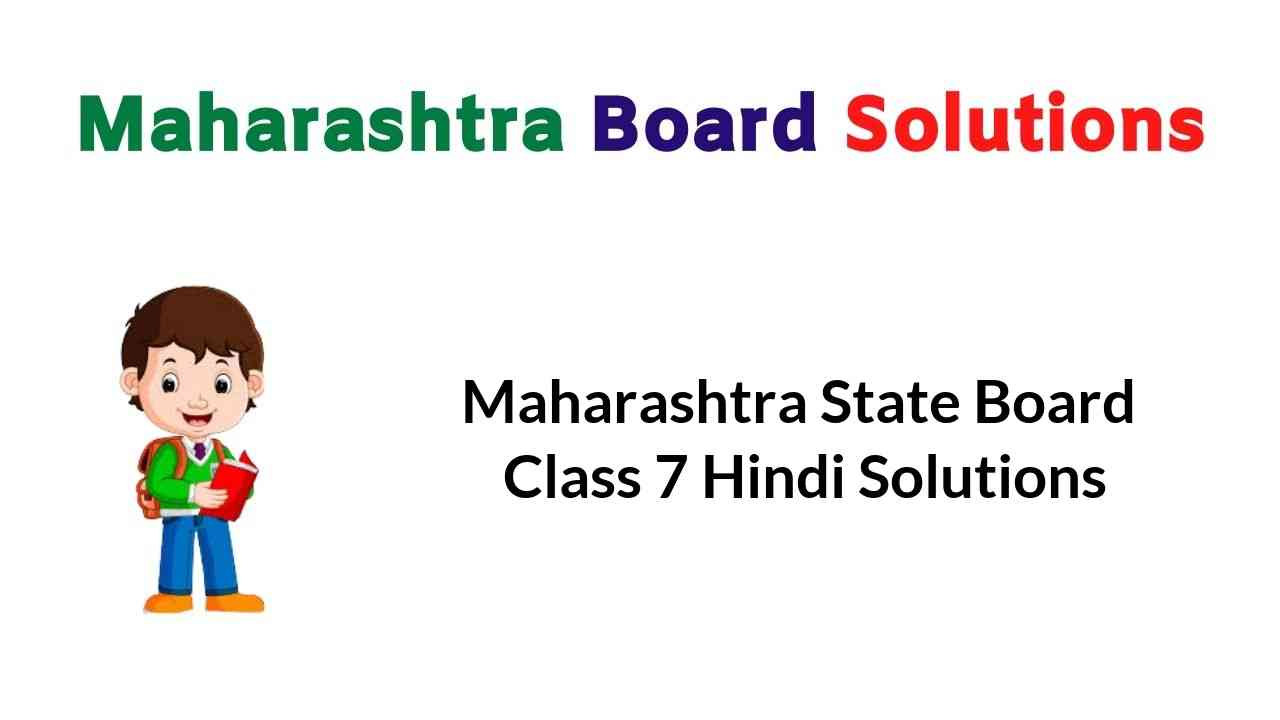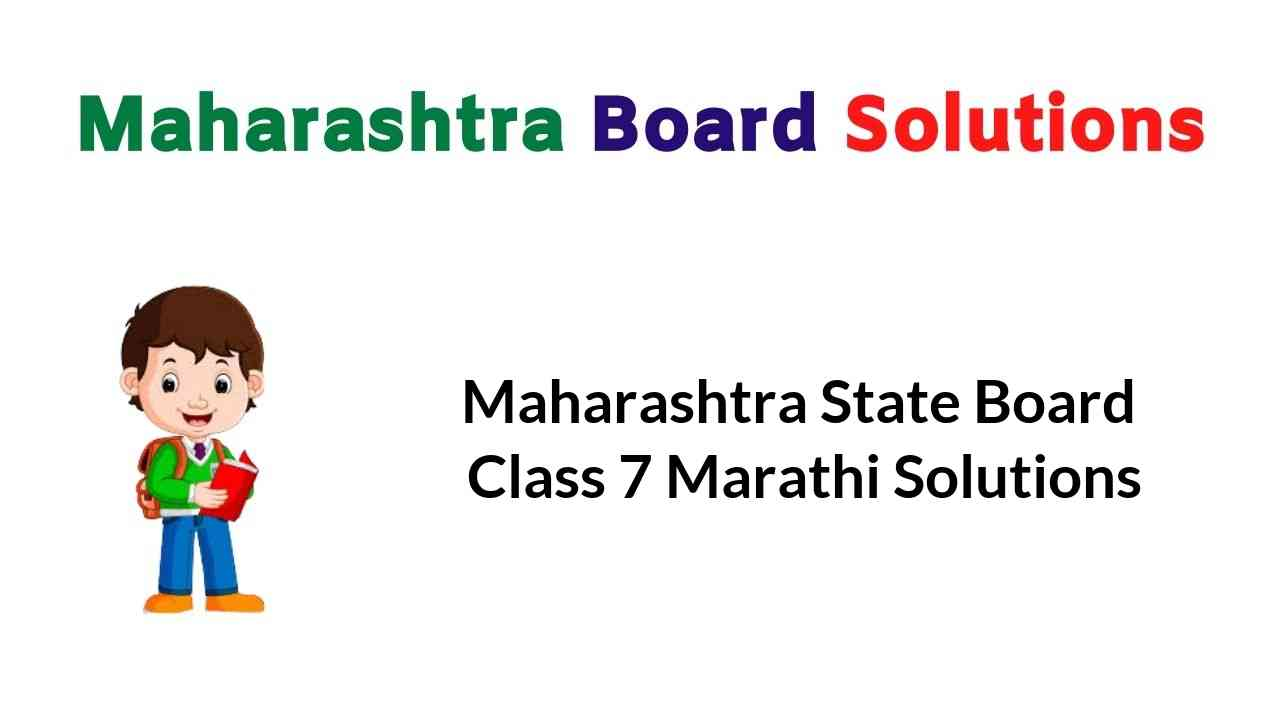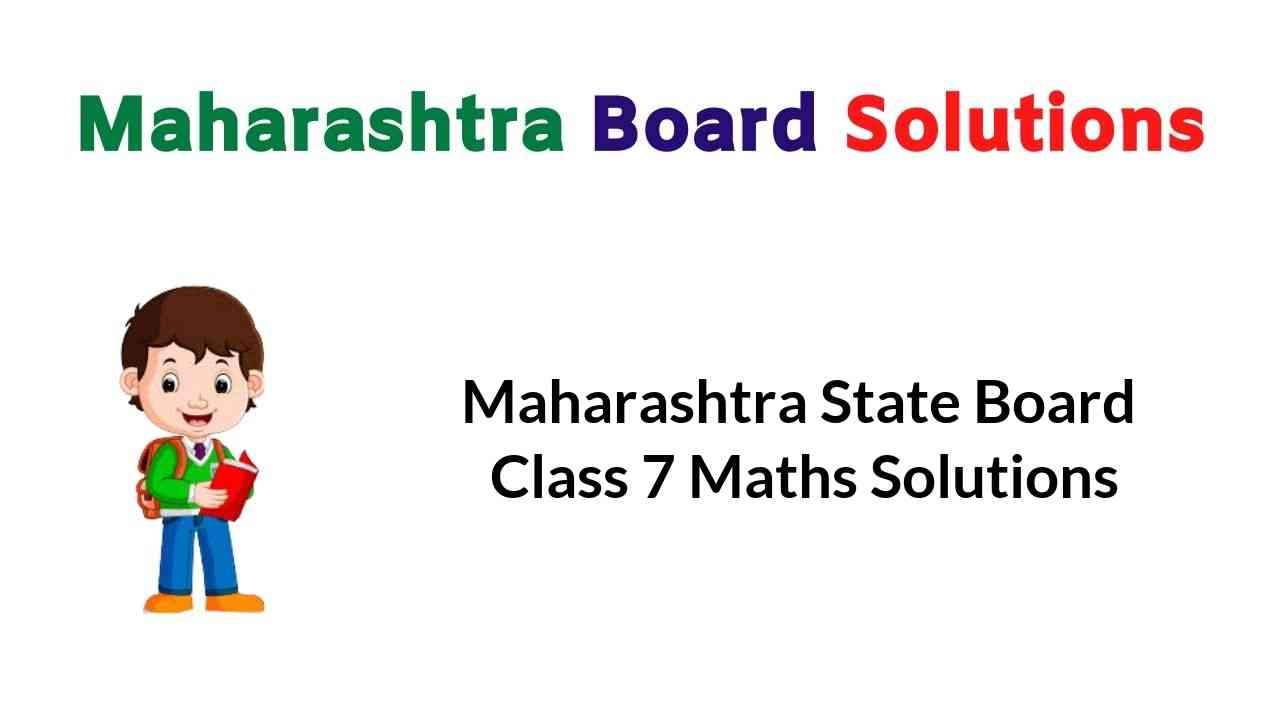## Maharashtra State Board 7th Std English Balbharati Textbook Solutions Answers Digest

English Balbharati Std 7 Digest Answers Unit 1

English Solution Class 7 Maharashtra Board Unit 2

English Balbharati Std 7 Digest Pdf Download Maharashtra Board Solutions Unit 3

7th Standard English Workbook Answers Maharashtra Board Unit 4

Maharashtra State Board Class 7 Textbook Solutions## Hindi Digest Std 7 Maharashtra Board | 7th Std Hindi Digest Pdf

7th Standard Hindi Digest Pdf | Std 7 Hindi Digest Pdf

Hindi Digest Std 7 Maharashtra Board पहली इकाई

7th Std Hindi Digest Pdf दूसरी इकाई

Maharashtra State Board Class 7 Textbook Solutions## Sulabhbharati 7th Class Marathi Textbook Solutions Answers Digest

Maharashtra State Board 7th Std Marathi Sulabhbharati Textbook Solutions

Marathi Sulabhbharati Class 7 Solutions

Maharashtra State Board Class 7 Textbook Solutions## Balbharati Solutions for Class 7 Maths | Maths Digest Std 7 Maharashtra State Board

Expert Teachers has created Maharashtra State Board Class 7 Maths Solutions Digest Pdf Download. You can also Download 7th Class Maths Book Solutions Maharashtra Board to help you to revise the complete Syllabus and score more marks in your examinations.

## Maths Solution Class 7 Maharashtra Board | 7th Standard Maths Digest

7th Std Maths Solution Maharashtra Board Chapter 1 Geometrical Constructions

Practice Set 7th Class Maharashtra State Board Chapter 2 Multiplication and Division of Integers

Class 7 Maths Solution Maharashtra Board Chapter 3 HCF and LCM

Class 7 Maths State Board Chapter 4 Angles and Pairs of Angles

Std 7 Maths Textbook Maharashtra Board Chapter 5 Operations on Rational Numbers

Class 7 Maths Maharashtra Board Chapter 6 Indices

Std 7 Maths Maharashtra Board Chapter 7 Joint Bar Graph

7th Maths Practice Set 32, 33, 34, 35 and 36 Chapter 8 Algebraic Expressions and Operations on them

Maharashtra Board Class 7 Maths Miscellaneous Problems Set 1

7th Standard Practice Set 37, 38 and 39 Direct Proportion and Inverse Proportion Chapter 9

Bank and Simple Interest Std 7 Practice Set 40 and 41 Chapter 10

7th Standard Maths Practice Set 42 and 43 Chapter 11 Circle

Class 7 Math Book Solution Chapter 12 Perimeter and Area

Maharashtra State Board Class 7 Maths Chapter 13 Pythagoras Theorem

Std 7 Maths Practice Set Chapter 14 Algebraic Formulae – Expansion of Squares

7th Standard Maths Maharashtra Board Chapter 15 Statistics

Maharashtra Board Class 7 Maths Miscellaneous Problems Set 2

Maharashtra State Board Class 7 Textbook Solutions

## Maharashtra Board Practice Set 40 Class 7 Maths Solutions Chapter 10 Bank and Simple Interest

Balbharti Maharashtra State Board Class 7 Maths Solutions covers the 7th Std Maths Practice Set 40 Answers Solutions Chapter 10 Bank and Simple Interest.

## Bank and Simple Interest Class 7 Practice Set 40 Answers Solutions Chapter 10

Question 1.
If Rihanna deposits Rs 1500 in the school fund at 9 p.c.p.a for 2 years, what is the total amount she will get?
Solution:
Here, P = Rs 1500, R = 9 p.c.p.a , T = 2 years
∴ Total interest = $$\frac{\mathrm{P} \times \mathrm{R} \times \mathrm{T}}{100}$$
= $$\frac{1500 \times 9 \times 2}{100}$$
= 1500 x 9 x 2
= Rs 270
∴ Total amount = Principal + Interest
= 1500 + 270
= Rs 1770
∴ Rihanna will get a total amount of Rs 1770.

Question 2.
Jethalal took a housing loan of Rs 2,50,000 from a bank at 10 p.c.p.a. for 5 years. What is the yearly interest he must pay and the total amount he returns to the bank?
Solution:
Here, P = Rs 250000, R = 10 p.c.p.a., T = 5 years
∴ Total interest = $$\frac{\mathrm{P} \times \mathrm{R} \times \mathrm{T}}{100}$$
= $$\frac{250000 \times 10 \times 5}{100}$$
= 2500 x 10 x 5
= Rs 1,25,000
∴ Yearly interest = Total interest ÷ Time = 1,25,000 ÷ 5 = Rs 25000
Total amount to be returned = Principal + Total interest
= 250000 + 125000 = Rs 375000
∴ The yearly interest is Rs 25,000 and Jethalal will have to return Rs 3,75,000 to the bank.

Question 3.
Shrikant deposited Rs 85,000 for $$2\frac { 1 }{ 2 }$$ years at 7 p.c.p.a. in a savings bank account. What is the total
interest he received at the end of the period?
Solution:
Here, P = Rs 85000, R = 7 p.c.p.a., T = $$2\frac { 1 }{ 2 }$$ years = 2.5 years
∴ Total interest = $$\frac{\mathrm{P} \times \mathrm{R} \times \mathrm{T}}{100}$$
= $$\frac{85000 \times 7 \times 2.5}{100}$$
= $$\frac{85000 \times 7 \times 25}{100 \times 10}$$
= 85 x 7 x 25
= Rs 14875
∴ The total interest received by Shrikant at the end of the period is Rs 14875.

Question 4.
At a certain rate of interest, the interest after 4 years on Rs 5000 principal is Rs 1200. What would be the interest on Rs 15000 at the same rate of interest for the same period?
Solution:
The interest on Rs 5000 after 4 years is Rs 1200.
Let us suppose the interest on Rs 15000 at the same rate after 4 years is Rs x.
Taking the ratio of interest and principal, we get
∴ $$\frac{x}{15000}=\frac{1200}{5000}$$
∴ $$x=\frac{1200 \times 15000}{5000}$$
= Rs 3600
∴ The interest received on Rs 15000 is Rs 3600.

Question 5.
If Pankaj deposits Rs 1,50,000 in a bank at 10 p.c.p.a. for two years, what is the total amount he will get from the bank?
Solution:
Here, P = 150000, R = 10 p.c.p.a., T = 2 years
∴ Total interest = $$\frac{\mathrm{P} \times \mathrm{R} \times \mathrm{T}}{100}$$
= $$\frac{150000 \times 10 \times 2}{100}$$
= Rs 30000
∴ Total amount = Principal + Total Interest
= 150000 + 30000
= Rs 180000
∴ Pankaj will receive Rs 180000 from the bank.

Maharashtra Board Class 7 Maths Chapter 10 Banks and Simple Interest Practice Set 40 Intext Questions and Activities

Question 1.
Observe the entries made in the page of a passbook shown below and answer the following questions. (Textbook pg. no. 70)1. On 2.2.16 the amount deposited was Rs__and the balance Rs__.
2. On 12.2.16, Rs__were withdrawn by cheque no. 243965. The balance was Rs__
3. On 26.2.2016 the bank paid an interest of Rs__

Solution:

1. 1500, 7000
2. 3000, 9000
3. 135

Practice Set 40 Class 7 Question 2.
Suvidya borrowed a sum of Rs 30000 at 8 p.c.p.a. interest for a year from her bank to buy a computer. At the end of the period, she had to pay back an amount of Rs 2400 over and above what she had borrowed.
Based on this information fill in the blanks below. (Textbook pg. no. 70)

1. Principal = Rs__
2. Rate of interest =__%
3. Interest = Rs__
4. Time =__year.
5. The total amount returned to the bank = 30,000 + 2,400 = Rs__

Solution:

1. 30000
2. 8
3. 2400
4. 1
5. Rs 32400